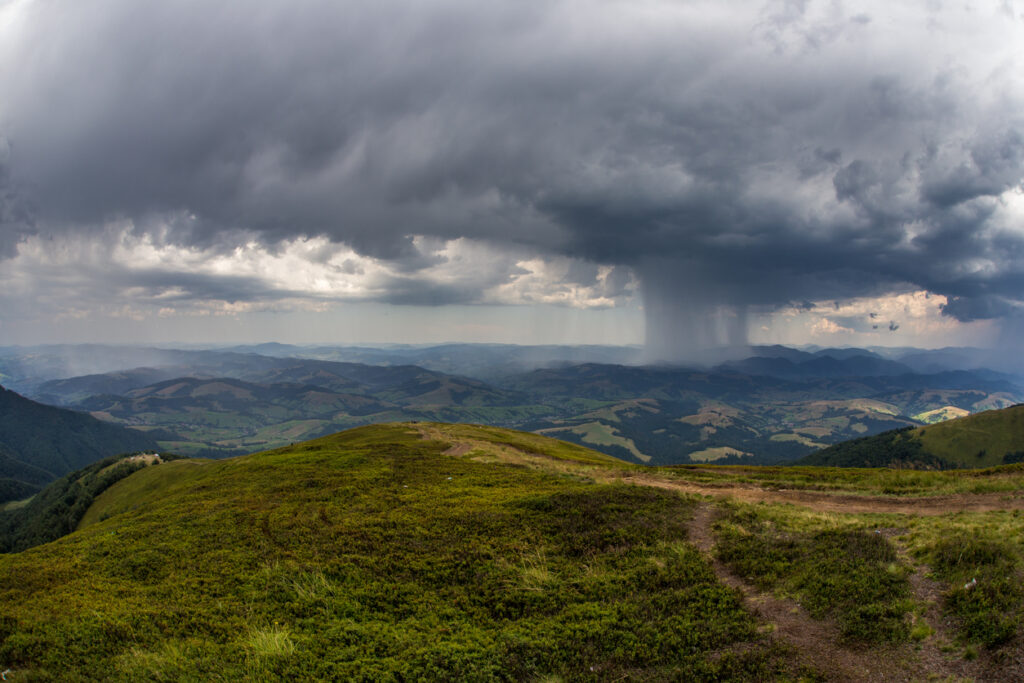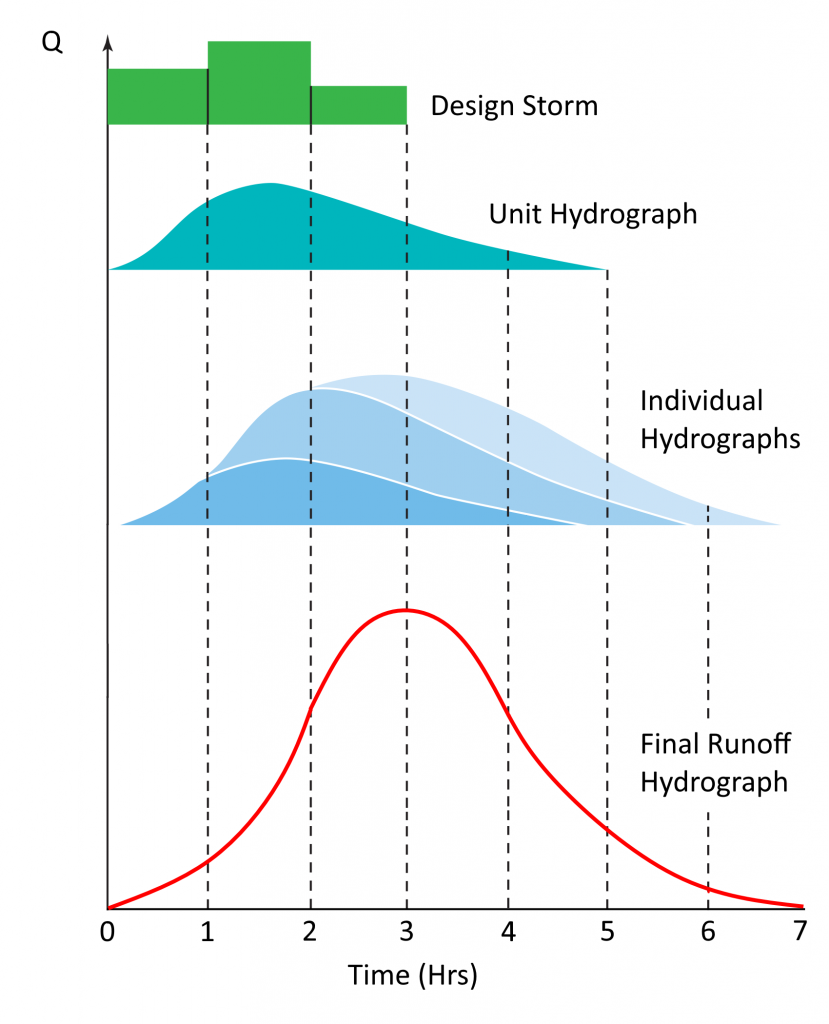# How SCS (NRCS) Hydrographs Are Made

This article explains exactly how the NRSC hydrographs are constructed in Hydrology Studio and hopefully will give you a better understanding of what goes on behind the scenes of the software. If you are looking for the procedure on how to add NRCS Hydrographs to your Basin Model, visit this page instead.A small portion of the precipitation from this storm will infiltrate into the soil. The rest will soon become a direct runoff hydrograph.

There are four steps involved in calculating NRCS runoff hydrographs. This method is the same as used in the original SCS TR-20.

1. A Design Storm is selected (Typically an NRCS 24-hr distribution).
2. A Unit Hydrograph is constructed based on the drainage area’s characteristics, i.e., Curve Number (CN), Tc, etc.
3. The Design Storm is applied to the Unit Hydrograph by multiplying each ordinate of the Design Storm (after it has been converted to an excess hyetograph) by each ordinate of the Unit Hydrograph, creating a series of Individual Hydrographs. A lot of them. For example, using a 1-minute Time Interval produces 1,440 individual Hydrographs. Each produced from the rainfall during the specified Time Interval.
4. The Individual Hydrographs are summed to create the Final Runoff Hydrograph.The Final Runoff Hydrograph is the summation of the Individual Hydrographs that were created by multiplying the ordinates in the Design Storm by each ordinate in the Unit Hydrograph.

## NRCS Unit Hydrograph

A unit hydrograph is a hydrograph resulting from 1 inch of rainfall excess on a watershed over a given time interval, for example, 1 minute. It is not the final runoff hydrograph but reflects the watershed’s unique characteristics. Think of the unit hydrograph like a person’s DNA. Once a unit hydrograph of a particular watershed is known, any design storm can be applied to it for computing the final runoff hydrograph.

The peak discharge for the Unit Hydrograph is computed as:

Where:
Qp = peak outflow, cfs (cms)
484 = Shape Factor (0.208 metric)*
A = catchment area, sq. miles (sq kilometers)
Q = total excess precipitation, 1 inch (1 mm)
Tp = time to peak (hrs)

* The shape factor is a user-definable variable. The default value is set to 484 and creates a unit hydrograph that has 3/8 of its area under its rising limb. This factor is higher in mountainous watersheds, for example, 600 (0.258), while in flat, sandy areas, will be lower, around 300 (0.129). The Delmarva peninsula in Delaware uses 284 (0.122) as does Maryland and Virginia. Michigan DOT uses a shape factor of 368 so that 28.5 percent of its volume is under the rising limb. This method is referred to as the MDEQ-SCS Method.

Related article: NRCS Unit Hydrograph Shape Factors

The Time to Peak, Tp, and the Time Base, Tb, are what determines the characteristics of the Unit Hydrograph. These values are computed as follows:

Where:
Tp = time to peak (hrs)
Tc = time of concentration (hrs)
D = time interval (hrs)
Tc = 1.67 x Lag Time (L)

Where:
L = lag time (hrs)
l = hydraulic length (ft)
S = (1000 / CN) – 10
Y = basin slope (%)
CN = curve number

Time Base = 2.67Tp

Where:
Tb = time base (hrs)
Tp = time to peak (hrs)

It should be noted that the program will adjust the Time to Peak so that it coincides with the current Time Interval. The smaller the Time Interval, (D) the more accurate the results.

## NRCS Design Storms

Hydrology Studio offers several built-in design storms including those which you can customize. Most of which are the NRCS 24-hr and 6-hr standard distributions and the latest distributions from NOAA Atlas 14. But other options include the IDF-based Synthetic “Balanced” Storms, Huff and as many as ten custom storms that you can input directly.

### NRCS 24-Hour Distributions

Hydrology Studio provides the full library of NRCS 24-hr as well as the 6-hour standard dimensionless distributions. The incremental rainfall amounts for the original SCS 24-hour storms are computed from polynomial equations which use coefficients that vary throughout the storm. The equation is of the form:

Where:
Pt = fraction of 24-hour precipitation
T = elapsed time (hrs)
C0 = coefficient
C1 = coefficient
C2 = coefficient
C3 = coefficient

Use of a polynomial-based design storm offers more accuracy than the original Tabular Method and as a result, you may experience slightly higher peak Qs, especially at small Tc’s.

### Synthetic (Nested or Balanced) Storms

Many practicing civil engineers use the NRCS 24-hour storms but keep in mind that any storm of any duration can be used with the unit hydrograph method. The Bulletin 75 Huff and the built-in synthetic, nested (Chicago) distributions are examples.

The Synthetic Storm option can actually produce an infinite number of design storm hyetographs but for practical reasons, Hydrology Studio limits them to 1, 2, 3, 6, 12 and 24-hour durations. The program uses your rainfall IDF curves to compute depth increments over the time intervals. From this, the design storm is constructed by placing the maximum depth increment near the center of the storm and arranging the other increments in a symmetrical alternating form. This is also known as a “Balanced Storm”.

This is the same method used by the SCS years ago to construct their 24-hour storms.

There’s much debate about the usefulness of a 24-hour storm applied to a small urban-like site with Tc’s as low as 15 minutes. The Synthetic Storm offers a good solution in that it can be matched to the site, that is, its total duration can be specified so that it better fits the computed Tc. For example, if Tc is 15 minutes, you could specify a one-hour storm rather than deal with a 24-hr storm. The one-hour storm lasts long enough so that the entire drainage area contributes to flow to the most downstream point. Going beyond Tc only adds unnecessary volume and calculation resources. A good rule of thumb for selecting a total storm duration is about 4 to 5 times Tc.

## Converting the Design Storm to Actual Runoff

Regardless of which Design Storm Distribution you are using, including the NRCS, Synthetic, Huff and the Custom storms directly input, the precipitation increments must be converted to excess precipitation (actual runoff). This is where the Curve Number comes in and determines how much of the actual rain is is lost due to abstractions, soil infiltration , etc., and how much becomes runoff or excess. The following equation is used:Where:
Q = excess volume of precipitation (in)
P = accumulated precipitation (in)
S = potential maximum retention
= (1000 / CN) – 10
CN = curve number

The computed volumes are then converted to excess increments used for the final excess precipitation “hyetograph”. It is this hyetograph that is used to form the final runoff hydrograph.

## Computing the Final Runoff Hydrograph

Hydrology Studio computes NRCS Method runoff hydrographs by convoluting a rainfall hyetograph through a unit hydrograph. This method is known as linear super positioning, and means that each ordinate of the rainfall design storm is multiplied by each ordinate of the unit hydrograph, thus creating a series of smaller hydrographs, each corresponding to a single rainfall amount over the specified Time Interval. These hydrographs are then summed to form the final runoff hydrograph. See illustration above.